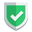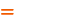我们相信：世界是美好的，你是我也是。平行空间的世界里面，不同版本的生活也在继续...

`Bunch`英文的解释是“束; 扎; 大量”等意思，当然，它作为`sklearn`里面的自定义数据类型。目前，还不知道其正式的类型中文翻译是什么。`Bunch`类型是基于`dict`字典类型的，所以，两者有何异同呢？

## `Bunch`类型定义

`sklearn`中的该类型的定义文件是：

``<python>\Lib\site-packages\sklearn\utils\_bunch.py``

``````class Bunch(dict):
def __init__(self, **kwargs):
super().__init__(kwargs)

def __setattr__(self, key, value):
self[key] = value

def __dir__(self):
return self.keys()

def __getattr__(self, key):
try:
return self[key]
except KeyError:
raise AttributeError(key)

def __setstate__(self, state):
pass``````

## `Bunch`和`dict`的不同之处

``````from sklearn.utils import Bunch
x1 = Bunch(name="苏南", name2="大叔")
x2 = {"name":"苏南", "name2":"大叔"} # dict
print(type(x1),type(x2))
print(x1.name,x1.name2)
print(x1["name"],x1["name2"])
print(x2["name"],x2["name2"])
# print(x2.name,x2.name2)       #  'dict' object has no attribute 'name'``````

• `Bunch``key`不用双引号，和`value`之间使用等号连接。
• `dict``key`必须使用双引号括上，和`value`之间使用冒号连接。

• `Bunch`可以使用`.attr`访问数据，还可以使用`["attr"]`访问数据。
• `dict`类型只可以使用`["attr"]`才能访问数据。

## `Bunch`和`dict`的相同之处

``````T = Bunch
t1 = T(left=T(left="苏南大叔",right="sunan大叔"),right=T(left="苏南同学",right="sunan同学"))
t2 = {"left":{"left":"苏南大叔","right":"sunan大叔"},"right":{"left":"苏南同学","right":"sunan同学"}}
print(t1.left)
print(t1.left.right)
print(t1['left']['right'])
print('left' in t1.right)
print('sunan同学' in t1.right)

print(t2["left"])
print(t2["left"]["right"])
print(t2['left']['right'])
print('left' in t2["right"])
print('sunan同学' in t2["right"])``````

## 总结如果本文对您有帮助，或者节约了您的时间，欢迎打赏瓶饮料，建立下友谊关系。本博客不欢迎：各种镜像采集行为。请尊重原创文章内容，转载请保留作者链接。【福利】 腾讯云最新爆款活动！1核2G云服务器首年50元！【源码】本文代码片段及相关软件，请点此获取更多信息【绝密】秘籍文章入口，仅传授于有缘之人python    sklearn